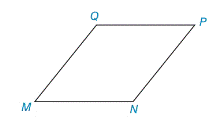Chapter 8.1, Problem 5E### Elementary Geometry for College St...

6th Edition
Daniel C. Alexander + 1 other
ISBN: 9781285195698

#### Solutions

Chapter
Section### Elementary Geometry for College St...

6th Edition
Daniel C. Alexander + 1 other
ISBN: 9781285195698
Textbook Problem
1 views

# In rhombus MNPQ, how does the length of the altitude from Q to P N ¯ compare to the length of the altitude from Q to M N ¯ ? Explain.Exercises 4–6

To determine

To Explain:

In rhombus MNPQ, the length of the altitude from Q to PN¯ and comparing it to the length of the altitude from Q to MN¯.

Explanation

Given,

In rhombus MNPQ,

The length of the altitude from Q to PN¯ is QR¯ and to the length of the altitude from Q to MN¯ is QS¯.

Find QR:

For the RPQ,

Here, PQ=x and RP=x2

By the Pythagorean theorem,

(RP)2+(QR)2=(PQ)2

(x2)2+(QR)2=(x)2

x24+(QR)2=(x)2

x24+(QR)2x24=x2x24

(QR)2=4x2x24

(QR)2=3x24

QR=3x24

QR=32x

Fi

### Still sussing out bartleby?

Check out a sample textbook solution.

See a sample solution

#### The Solution to Your Study Problems

Bartleby provides explanations to thousands of textbook problems written by our experts, many with advanced degrees!

Get Started

#### Simplify: 918

Elementary Technical Mathematics

#### In Exercises 1-22, evaluate the given expression. C(7,5)

Finite Mathematics for the Managerial, Life, and Social Sciences

#### True or False: y′ + xey = ex+y is separable.

Study Guide for Stewart's Single Variable Calculus: Early Transcendentals, 8th

#### It does not exist.

Study Guide for Stewart's Multivariable Calculus, 8th